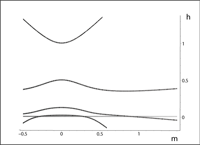July 2011
Mon Tue Wed Thu Fri Sat Sun
« Jun   Aug »
123
45678910
11121314151617
18192021222324
25262728293031

## Spectral Singularities of a Complex Spherical Barrier Potential and Their Optical Realization

Ali Mostafazadeh, Mustafa SarisamanThe mathematical notion of a spectral singularity admits a physical interpretation as a zero-width resonance. It finds an optical realization as a certain type of lasing effect that occurs at the threshold gain. We explore spectral singularities of a complex spherical barrier potential and study their realization as transverse spherical electromagnetic waves emitted by a gain medium with a spherical geometry. In particular, for a typical dye laser material, we obtain a lower bound on the size of the gain medium for the occurence of this kind of spectral singularities.

http://arxiv.org/abs/1107.1873
Mathematical Physics (math-ph); Optics (physics.optics); Quantum Physics (quant-ph)

## The cryptohermitian smeared-coordinate representation of wave functions

Miloslav ZnojilThe one-dimensional real line of coordinates is replaced, for simplification or approximation purposes, by an N-plet of the so called Gauss-Hermite grid points. These grid points are interpreted as the eigenvalues of a tridiagonal matrix $$\mathfrak{q}_0$$ which proves rather complicated. Via the “zeroth” Dyson-map $$\Omega_0$$ the “operator of position” $$\mathfrak{q}_0$$ is then further simplified into an isospectral matrix $$Q_0$$ which is found optimal for the purpose. As long as the latter matrix appears non-Hermitian it is not an observable in the manifestly “false” Hilbert space $${\cal H}^{(F)}:=\mathbb{R}^N$$. For this reason the optimal operator $$Q_0$$ is assigned the family of its isospectral avatars $$\mathfrak{h}_\alpha$$, $$\alpha=(0,)\,1,2,…$$. They are, by construction, selfadjoint in the respective $$\alpha-$$dependent image Hilbert spaces $${\cal H}^{(P)}_\alpha$$ obtained from $${\cal H}^{(F)}$$ by the respective “new” Dyson maps $$\Omega_\alpha$$. In the ultimate step of simplification, the inner product in the F-superscripted space is redefined in an {\it ad hoc}, $\alpha-$dependent manner. The resulting “simplest”, S-superscripted representations $${\cal H}^{(S)}_\alpha$$ of the eligible physical Hilbert spaces of states (offering different dynamics) then emerge as, by construction, unitary equivalent to the (i.e., indistinguishable from the) respective awkward, P-superscripted and $$\alpha-$$subscripted physical Hilbert spaces.

http://arxiv.org/abs/1107.1770
Quantum Physics (quant-ph); Mathematical Physics (math-ph)

## Imaginary-Scaling versus Indefinite-Metric Quantization of the Pais-Uhlenbeck Oscillator

Ali Mostafazadeh

Using the Pais-Uhlenbeck Oscillator as a toy model, we outline a consistent alternative to the indefinite-metric quantization scheme that does not violate unitarity. We describe the basic mathematical structure of this method by giving an explicit construction of the Hilbert space of state vectors and the corresponding creation and annihilation operators. The latter satisfy the usual bosonic commutation relation and differ from those of the indefinite-metric theories by a sign in the definition of the creation operator. This change of sign achieves a definitization of the indefinite-metric that gives life to the ghost states without changing their contribution to the energy spectrum.

http://arxiv.org/abs/1107.1874
High Energy Physics – Theory (hep-th); General Relativity and Quantum Cosmology (gr-qc); Mathematical Physics (math-ph); Quantum Physics (quant-ph)

## Edge states and topological phases in non-Hermitian systems

Kenta Esaki, Masatoshi Sato, Kazuki Hasebe, Mahito KohmotoTopological stability of the edge states is investigated for non-Hermitian systems. We examine two classes of non-Hermitian Hamiltonians supporting real bulk eigenenergies in weak non-Hermiticity: SU(1,1) and SO(3,2) Hamiltonians. As an SU(1,1) Hamiltonian, the tight-binding model on the honeycomb lattice with imaginary on-site potentials is examined. Edge states with ReE=0 and their topological stability are discussed by the winding number and the index theorem, based on the pseudo-anti-Hermiticity of the system. As a higher symmetric generalization of SU(1,1) Hamiltonians, we also consider SO(3,2) models. We investigate non-Hermitian generalization of the Luttinger Hamiltonian on the square lattice, and that of the Kane-Mele model on the honeycomb lattice, respectively. Using the generalized Kramers theorem for the time-reversal operator Theta with Theta^2=+1 [M. Sato et al., arXiv:1106.1806], we introduce a time-reversal invariant Chern number from which topological stability of gapless edge modes is argued.

http://arxiv.org/abs/1107.2079
Mesoscale and Nanoscale Physics (cond-mat.mes-hall); Other Condensed Matter (cond-mat.other)

## Spectral Singularities of a General Point Interaction

Ali MostafazadehWe study the problem of locating spectral singularities of a general complex point interaction with a support at a single point. We also determine the bound states, examine the special cases where the point interaction is P-, T-, and PT-symmetric, and explore the issue of the coalescence of spectral singularities and bound states.

http://arxiv.org/abs/1107.1875
Mathematical Physics (math-ph); Quantum Physics (quant-ph)